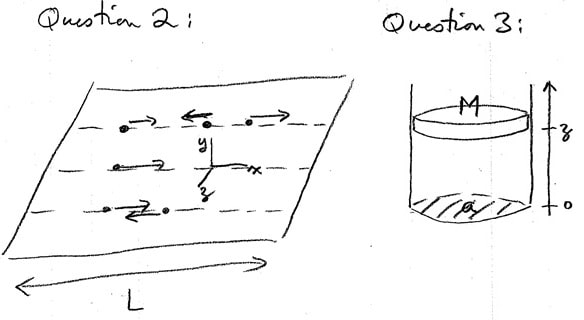# PHY5355 assignment 2

1. Paramagnetism for spin s — 1/2 case, using microcanonical ensemble: Consider N distinguishable, non-interacting spins in a magnetic field H. Each spin has magnetic moment of size ,u, and can point either parallel or antiparallel to H. The energy of a particular microstate is therefore (—ni/JH) where ni = (a) Find the number p (N, H, E) of microstates consistent with the macroscopic parameters given. (b) Using (a), derive the entropy S(N, H, E) in the thermodynamic limit. (c) Using (b), find the temperature T (N, H, E), and hence internal energy U (N, H, T) and magnetization (i.e. net magnetic moment of system) M (N, H, T). (c) Determine the T -+ 0 behaviour of U and S.
2. A surface of a solid has R rows of length L at which gas atoms can adsorb. Suppose the gas atoms in a given row move freely in the x-direction, but are constrained in the y and z directions by the harmonic potential U (F) 1 (Y2 + z2 ) Eo where the binding energy is Eo > 0. The surface is in equilibrium with a (3-d) gas phase. Derive the number of adsorbed atoms per unit length per row, as a function of the (3-d) gas pressure P. (Ignore interactions between all gas atoms with each other.) Use the grand canonical ensemble.
3. Consider N noninteracting gas atoms in a container of cross-sectional area The top of the container is an air-tight piston of mass M which slides frictionlessly. (a) Construct the partition function Q of the N + 1 particle system. (N gas atoms of mass m and one piston of mass M.) You may neglect the effect of gravity on the gas atoms, and ignore atmospheric pressure outside the container. The piston has only one degree of freedom. (b) Obtain the Helmholtz free energy A of the piston+gas system, in the thermodynamic limit. Show that it is identical to the Gibb's free energy G(P, N, T) for a system of N particles of mass m at temperature T and pressure P = Mg/a.